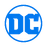## FANDOM

110,207 PagesThis page contains a listing of all known appearances of Garryn Bek (New Earth).
If you find an Issue this Character appears in that is not shown here, please edit that issue adding "Garryn Bek (New Earth)" as a Cast member.
See Also: The Garryn Bek (New Earth) gallery

All items (76)

A
•Adventures of Superman Annual Vol 1 2
D
•Darkstars Vol 1 11
•DC Comics Encyclopedia
•DC Comics Encyclopedia: All-New Edition
•DC Comics Encyclopedia: Updated and Expanded
•DCU: Trinity Vol 1 2
G
•Green Lantern Vol 3 45
I
•Infinite Crisis Vol 1 1
•Infinite Crisis Special: Rann-Thanagar War Vol 1 1
•Invasion! Vol 1 1
•Invasion! Vol 1 2
L
•L.E.G.I.O.N. Vol 1 1
•L.E.G.I.O.N. Vol 1 2
•L.E.G.I.O.N. Vol 1 3
•L.E.G.I.O.N. Vol 1 4
•L.E.G.I.O.N. Vol 1 5
•L.E.G.I.O.N. Vol 1 6
•L.E.G.I.O.N. Vol 1 7
•L.E.G.I.O.N. Vol 1 8
•L.E.G.I.O.N. Vol 1 9
•L.E.G.I.O.N. Vol 1 10
•L.E.G.I.O.N. Vol 1 11
•L.E.G.I.O.N. Vol 1 12
•L.E.G.I.O.N. Vol 1 13
•L.E.G.I.O.N. Vol 1 14
•L.E.G.I.O.N. Vol 1 15
•L.E.G.I.O.N. Vol 1 16
•L.E.G.I.O.N. Vol 1 17
•L.E.G.I.O.N. Vol 1 19
•L.E.G.I.O.N. Vol 1 20
•L.E.G.I.O.N. Vol 1 21
•L.E.G.I.O.N. Vol 1 22
•L.E.G.I.O.N. Vol 1 23
•L.E.G.I.O.N. Vol 1 24
•L.E.G.I.O.N. Vol 1 25
•L.E.G.I.O.N. Vol 1 26
•L.E.G.I.O.N. Vol 1 27
•L.E.G.I.O.N. Vol 1 29
•L.E.G.I.O.N. Vol 1 30
•L.E.G.I.O.N. Vol 1 34
•L.E.G.I.O.N. Vol 1 35
•L.E.G.I.O.N. Vol 1 36
•L.E.G.I.O.N. Vol 1 37
•L.E.G.I.O.N. Vol 1 38
•L.E.G.I.O.N. Vol 1 39
•L.E.G.I.O.N. Vol 1 40
•L.E.G.I.O.N. Vol 1 44
•L.E.G.I.O.N. Vol 1 45
•L.E.G.I.O.N. Vol 1 46
•L.E.G.I.O.N. Vol 1 47
•L.E.G.I.O.N. Vol 1 48
•L.E.G.I.O.N. Vol 1 49
•L.E.G.I.O.N. Vol 1 50
•L.E.G.I.O.N. Vol 1 52
•L.E.G.I.O.N. Vol 1 54
•L.E.G.I.O.N. Vol 1 56
•L.E.G.I.O.N. Vol 1 57
•L.E.G.I.O.N. Vol 1 58
•L.E.G.I.O.N. Vol 1 59
•L.E.G.I.O.N. Vol 1 60
•L.E.G.I.O.N. Vol 1 61
•L.E.G.I.O.N. Vol 1 62
•L.E.G.I.O.N. Vol 1 63
•L.E.G.I.O.N. Vol 1 64
•L.E.G.I.O.N. Vol 1 66
•L.E.G.I.O.N. Vol 1 67
•L.E.G.I.O.N. Vol 1 69
•L.E.G.I.O.N. Vol 1 70
•L.E.G.I.O.N. Annual Vol 1 2
•Lobo Vol 2 62
R
•R.E.B.E.L.S. Vol 1 7
•R.E.B.E.L.S. Vol 1 8
•R.E.B.E.L.S. Vol 1 16
V
•Valor Vol 1 4
•Valor Vol 1 12
W
•Who's Who in the DC Universe Vol 1 10
Community content is available under CC-BY-SA unless otherwise noted.

## Bring Your DC Movies Together